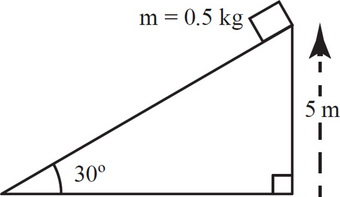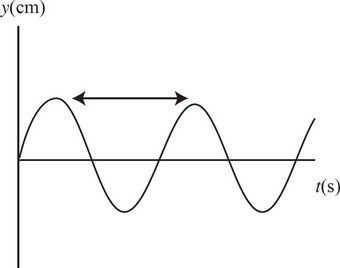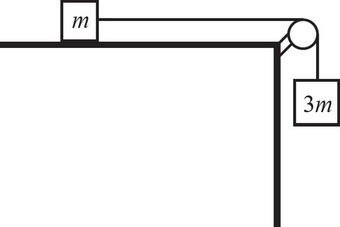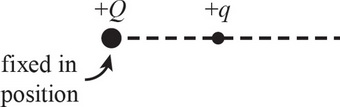# AP Physics 1 Practice Test 1

### Test Information10 questions18 minutes

1. What happens to the force of gravitational attraction between two small objects if the mass of each object is doubled and the distance between their centers is doubled?

2.If the surface of the incline is frictionless, how long will the block take to reach the bottom if it was released from rest at the top?

3.If the surface of the incline is frictionless, with what speed will the block reach the bottom if it was released from rest at the top?

4.If the coefficient of friction between the block and the incline is 0.4, how much work is done by the normal force on the block as it slides down the full length of the incline?

5. Two satellites are in circular orbit around the Earth. The distance from Satellite 1 to Earth’s center is r1, and the distance from Satellite 2 to the Earth’s center is r2, what is the speed of Satellite 2?

6.The graph above shows a wave. What quantity of the wave does the indicated segment represent?

7. Sound waves travel at 350 m/s through warm air and at 3,500 m/s through brass. What happens to the wavelength of a 700 Hz acoustic wave as it enters brass from warm air?

8.In the figure above, the coefficient of sliding friction between the small block and the tabletop is 0.2. If the pulley is frictionless and massless, what will be the acceleration of the blocks once they are released from rest?

9. Two people, one of mass 100 kg and the other of mass 50 kg, stand facing each other on an ice-covered (essentially frictionless) pond. If the heavier person pushes on the lighter one with a force F, then

10.The figure above shows two positively charged particles. The +Q charge is fixed in position, and the +Q charge is brought close to +Q and released from rest. Which of the following graphs best depicts the acceleration of the +Q charge as a function of its distance R from +Q ?